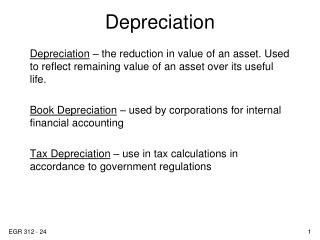DownloadDownload PresentationDepreciation

# Depreciation

Download Presentation## Depreciation

- - - - - - - - - - - - - - - - - - - - - - - - - - - E N D - - - - - - - - - - - - - - - - - - - - - - - - - - -
##### Presentation Transcript

1. Depreciation Depreciation – the reduction in value of an asset. Used to reflect remaining value of an asset over its useful life. Book Depreciation – used by corporations for internal financial accounting Tax Depreciation – use in tax calculations in accordance to government regulations

2. Why is depreciation important? Depreciation can lower your taxes: taxes = (income – deductions)*tax rate where one of the primary deductions is depreciation. In other words, the use of depreciation can make you money by reducing the amount of taxes you pay.

3. Definitions First Cost – cost of purchasing and installing an asset (on real-estate, the value of land is excluded) Book Value – the remaining, undepreciated capital of an asset which is on the corporation’s books; the first cost minus the sum of all deprecation taken Recovery Period – depreciable life of the asset in years Market Value – estimate of the value of an asset if sold on the open market, not necessarily the same as the book value.

4. Definitions Depreciation Rate – the fraction of the “First Cost” removed by depreciation each year. Personal Property – allowed for depreciation, includes items such as manufacturing equipment, vehicles, computers, etc. Real Property – also allowed for depreciation, includes office buildings, warehouses, manufacturing facilities, etc… note, land is not depreciated. Half-year convention – assumes assets are placed in service in midyear.

5. Straight Line (SL) Depreciation Book value depreciates linearly with time. In other words, depreciation is removed in equal amounts each year. Where t = year (1,2,… n) Dt= annual depreciation charge B = first cost S = estimated salvage value n = recovery period d = depreciation rate = 1/n

6. SL Depreciation Book value (SL): Depreciation rate is constant:

7. SL Depreciation Example: A \$20,000 vehicle is to be depreciated over 7 years using SL depreciation.

8. Depreciation Straight Line (SL) Depreciation Example: What would the yearly depreciation and depreciation rate be if the \$20,000 vehicle is expected to have a salvage value of \$6,000? Dt = _____________ d = ______________

9. Declining Balance (DB) Depreciation Book value depreciates by a fixed percentage of the book value, not a fixed amount. Where t = year (1,2,… n) BVt-1= book value in year t - 1 Dt = depreciation amount in year t d = depreciation rate

10. DB Depreciation Book value (DB):

11. DB Depreciation Example: A \$20,000 vehicle is to be depreciated over 7 years using DB depreciation with a depreciation rate of 0.25.

12. Double Declining Balance (DDB) Depreciation The maximum annual depreciation rate for DB method is: dmax = 2/n In this case, the method is called double declining balance (DDB)

13. Modified Accelerated Cost Recovery System (MACRS) MACRS is the US government accepted depreciation schedule for tax purposes. MACRS combines facets of DDB and SL methods. Assets are grouped into categories based on recovery periods of 3, 5, 7, 10, 15, 20, 27.5, and 39 years. See table 16-4, pg. 546 for asset groupings. Examples: landscaping around the UC rental house tooling for new line of refrigerators

14. MACRS To determine the amount of deprecation each year, use the following depreciation rate table (table 16-2, pg. 542.)

15. MACRS Example: A \$20,000 vehicle is to be depreciated for tax purposes.